2022 NFL Passing Leader Odds
+400
4 to 1Josh Allen
14.6% implied probability

+400
4 to 1Justin Herbert
14.6% implied probability

+600
6 to 1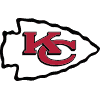Patrick Mahomes
10.4% implied probability

+1000
10 to 1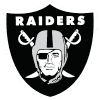Derek Carr
6.6% implied probability

+1000
10 to 1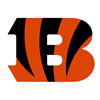Joe Burrow
6.6% implied probability

+1200
12 to 1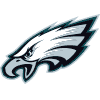Jalen Hurts
5.6% implied probability

+1600
16 to 1Matthew Stafford
4.3% implied probability

+1800
18 to 1Tom Brady
3.8% implied probability

+2000
20 to 1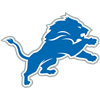Jared Goff
3.5% implied probability

+2200
22 to 1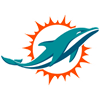Tua Tagovailoa
3.2% implied probability

+2500
25 to 1Carson Wentz
2.8% implied probability

+2500
25 to 1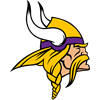Kirk Cousins
2.8% implied probability

+3000
30 to 1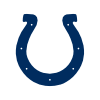Matt Ryan
2.4% implied probability

+3500
35 to 1Kyler Murray
2.0% implied probability

+4000
40 to 1Trevor Lawrence
1.8% implied probability

+5000
50 to 1Russell Wilson
1.4% implied probability

+5000
50 to 1Lamar Jackson
1.4% implied probability

+5000
50 to 1Jameis Winston
1.4% implied probability

+6000
60 to 1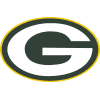Aaron Rodgers
1.2% implied probability

+6000
60 to 1Mac Jones
1.2% implied probability

+8000
80 to 1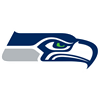Geno Smith
0.9% implied probability

+8000
80 to 1Dak Prescott
0.9% implied probability

+8000
80 to 1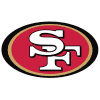Jimmy Garoppolo
0.9% implied probability

+10000
100 to 1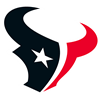Davis Mills
0.7% implied probability

+10000
100 to 1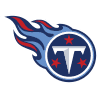Ryan Tannehill
0.7% implied probability

+15000
150 to 1Zach Wilson
0.5% implied probability

+15000
150 to 1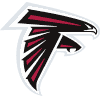Marcus Mariota
0.5% implied probability

+15000
150 to 1Joe Flacco
0.5% implied probability

+20000
200 to 1Teddy Bridgewater
0.4% implied probability

+20000
200 to 1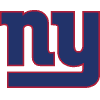Daniel Jones
0.4% implied probability

+20000
200 to 1Baker Mayfield
0.4% implied probability

+20000
200 to 1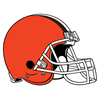Jacoby Brissett
0.4% implied probability

+25000
250 to 1Kenny Pickett
0.3% implied probability

+30000
300 to 1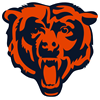Justin Fields
0.2% implied probability

+30000
300 to 1Mitch Trubisky
0.2% implied probability

+50000
500 to 1Drew Lock
0.1% implied probability

+50000
500 to 1Malik Willis
0.1% implied probability